JMSLTM Numerical Library 7.2.0
com.imsl.stat

## Class Difference

• All Implemented Interfaces:
Serializable, Cloneable

```public class Difference
extends Object
implements Serializable, Cloneable```
Differences a seasonal or nonseasonal time series.

Class `Difference` performs m = `periods.length` successive backward differences of period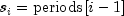and order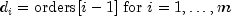on the n = `z.length` observations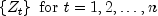.

Consider the backward shift operator B given byfor all k. Then, the backward difference operator with period s is defined by the following: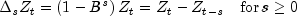Note that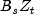and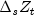are defined only for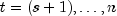. Repeated differencing with period s is simply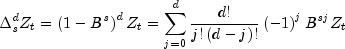where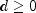is the order of differencing. Note that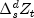is defined only for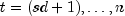.

The general difference formula used in the class `Difference` is given by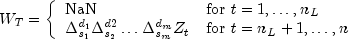where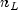represents the number of observations "lost" because of differencing and NaN represents the missing value code. Note that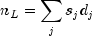A homogeneous, stationary time series can be arrived at by appropriately differencing a homogeneous, nonstationary time series (Box and Jenkins 1976, p. 85). Preliminary application of an appropriate transformation followed by differencing of a series can enable model identification and parameter estimation in the class of homogeneous stationary autoregressive moving average models.

Example 1, Example 2, Serialized Form
• ### Constructor Summary

Constructors
Constructor and Description
`Difference()`
Constructor for `Difference`.
• ### Method Summary

Methods
Modifier and Type Method and Description
`double[]` ```compute(double[] z, int[] periods)```
Computes a Difference series.
`void` `excludeFirst(boolean exclude)`
If set to true, the observations lost due to differencing will be excluded.
`int` `getObservationsLost()`
Returns the number of observations lost because of differencing the time series.
`void` `setOrders(int[] orders)`
Sets the orders for the Difference object
• ### Methods inherited from class java.lang.Object

`clone, equals, finalize, getClass, hashCode, notify, notifyAll, toString, wait, wait, wait`
• ### Constructor Detail

• #### Difference

`public Difference()`
Constructor for `Difference`.
• ### Method Detail

• #### compute

```public final double[] compute(double[] z,
int[] periods)
throws IllegalArgumentException```
Computes a Difference series.
Parameters:
`z` - a `double` array containing the time series.
`periods` - an `int` array containing the periods at which z is to be differenced.
Returns:
a `double` array containing the differenced series.
Throws:
`IllegalArgumentException`
• #### excludeFirst

`public void excludeFirst(boolean exclude)`
If set to true, the observations lost due to differencing will be excluded. The differenced series will be the length of the number of observations minus the number of observations lost. If set to false, the observations lost due to differencing will be set to NaN (Not a number) and included in the differenced series. The default is to set the lost observations to NaN.
Parameters:
`exclude` - a `boolean` specifying whether or not to exclude lost observations due to differencing.
• #### getObservationsLost

`public int getObservationsLost()`
Returns the number of observations lost because of differencing the time series. Note that the `compute` method must be invoked first before invoking this method. Otherwise, the return value is `0`.
Returns:
an `int` containing the number of observations lost because of differencing the time series `z`.
• #### setOrders

`public void setOrders(int[] orders)`
Sets the orders for the Difference object
Parameters:
`orders` - an `int` array of length equal to length of `periods`, containing the order of each difference given in periods. The elements of orders must be greater than or equal to 0.
JMSLTM Numerical Library 7.2.0